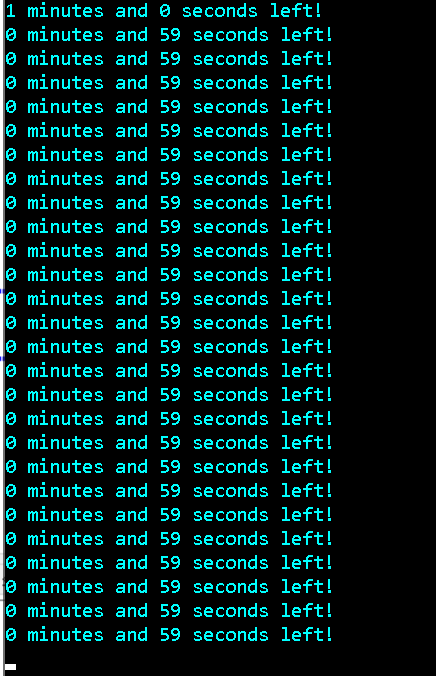Ask coding questions

← Back to all posts
##### Help - My code isn't working

So I'm trying to make a countdown, but this happensHere's my code, any help is appreciated.

``````#include <iostream>
#include <windows.h>

using namespace std;

int sec = 0;
int minu = 1;

void Seconds () {
bool stay = true;

while (minu > 0) {
cout << minu << " minutes and " << sec << " seconds left!" << endl;

if (sec == 0) {
sec = 60;
minu -= 1;
}

Sleep (1000);
sec -= 1;
}

if (minu == 0) {
while (sec > 0) {
Sleep (1000);
cout << minu << " minutes and " << sec << " seconds left!" << endl;
}

cout << "Time is up!" << endl;
}
}

int main () {
Seconds ();

return 0;
}``````

and yes, I am using a windows PC

Answered by ch1ck3n (1622) [earned 5 cycles]
View Answer
##### Comments
hotnewtop
ch1ck3n (1622)

What is wrong? what is the desired output?

Streakwind (0)

@ch1ck3n Nevermind, I forgot to -1 to seconds.